Browse by Topic
Related Topics

# What Shall We Do with the Drunken Sailor? Make Him Walk the Plank!

Back in 1988 when Mathematica was just a year old and no one in my university had heard of it, I was forced to learn Fortran.

My end-of-term project was this problem: “A drunken sailor returns to his ship via a plank 15 paces long and 7 paces wide. With each step he has an equal chance of stepping forward, left, right, or standing still. What is the probability that he returns safely to his ship?” I wrote a page or so of ugly code, passed the course, and never wrote Fortran again. Today I thought I would revisit the problem.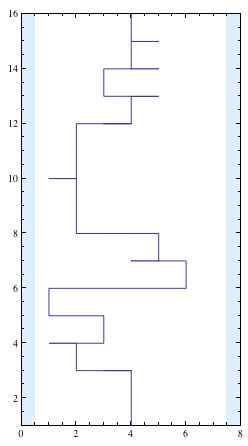We can code the logic of the sailor’s walk quite easily using separate rules for each case. Firstly, if he is ever on the 16th step or already on the ship, then he is safely on the ship the next time.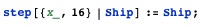If he is ever outside the bounds of the plank, at position 0 or 8, then he falls in the water and stays there: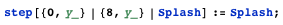And for all other cases, he steps to one of four new positions.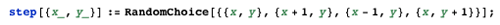To simulate an entire journey, we repeatedly step until he reaches one of the named locations.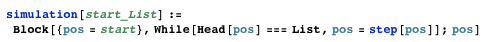We are now able to run a full simulation from a given start point. Let’s be kind to our sailor and have the concierge of the Monte Carlo Casino, where he has been drinking all night, place him in the middle of the end of the plank before leaving him. This is position {4, 1}.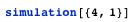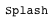Using FixedPointList instead of While gives a neat way to visualize his stagger.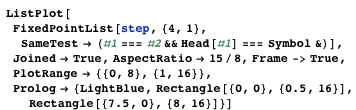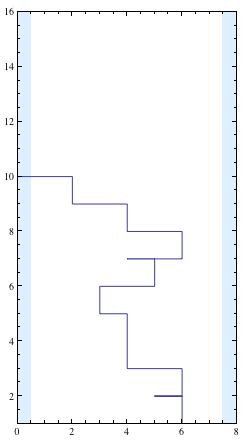Now all we need to do is to run the simulation lots of times and count the successes versus the failures: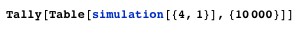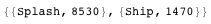This gives us about a 1470(1470+8530) probability of success, or 14.7%, and it took only five lines of code.

Because of the discrete-state nature of the problem, there is another way to look at it that efficiently gives us the probability not only of this journey, but the chances of getting from any one place to any other place in any specific number of steps, and that is to use Markov chains. I didn’t know anything about Markov chains in 1988, so I have no idea how much work it would have been in Fortran, but here is how you might do it in Mathematica.

First we number all of the possible positions. In the 15 × 7 problem there are 105 possible places to stand on the plank. I will designate the water as position 106 and ship as 107 (or more generally water is width × height + 1 and ship as width × height + 2). Now we construct a matrix to represent all the transition probabilities. The element Ma, b contains the probability that we will step from position a to position b in any one step. For example, since the chance of moving from position 1 to position 2 is ¼, M1, 2 = ¼, etc. If we do this right, every row of the transition matrix sums to 1 since there is a 100% probability that we go to some state. Because at any one time we can only step to 4 possible places out of the 107, the resulting matrix is quite sparse, and so using SparseArray will speed up the calculations.

Constructing the matrix is the trickiest part of the problem, but fortunately SparseArray has a rather neat pattern specification that allows us to describe all of the values in a few lines.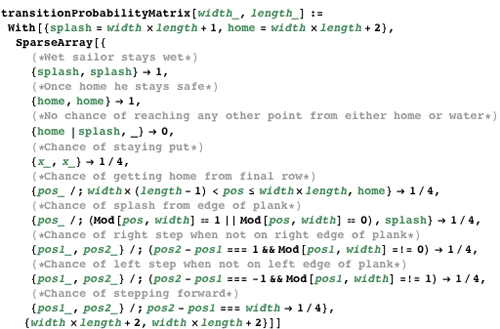Here for example is the transition matrix for a small 3 × 3 plank: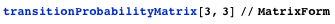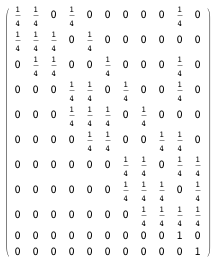And here is the transition matrix for our 7 × 15 problem; it has over 10,000 elements, yet all but 422 are zero, making it very efficient as a SparseArray. The nonzero elements follow a similar pattern to the 3 × 3 example.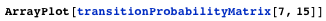Here is the first row describing the possible transitions from position 1 (the left-hand edge of the start of the plank). It shows equal chances of going to position 1 (standing still), 2 (stepping right), 8 (stepping forward), and 106 (splash).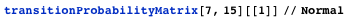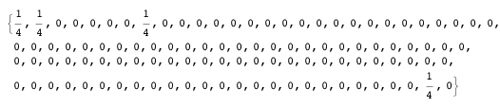The important thing about the transition matrix in Markov chains is that Mn represents the transition probability matrix for each start position to end position after exactly n steps. This is my very favorite use of matrices in problem solving. When I learned it, finding powers of matrices was hard work, involving decomposing them into diagonal matrices, but now I can just use MatrixPower. Because MatrixPower supports sparse methods, it is very fast on this problem.

So for example, here are the probabilities for where we will find the sailor who started in the middle of the plank after six steps, showing a 23512 chance (position -2) that he is swimming already.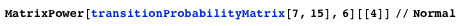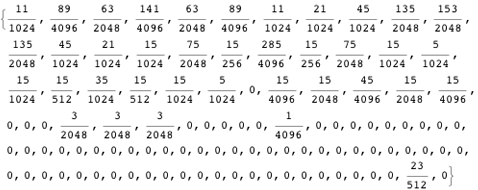This Manipulate lets you visualize his probable position (if still on the plank) along with numeric values of his probability of reaching each final destination.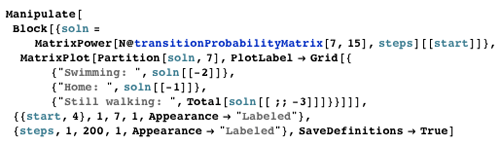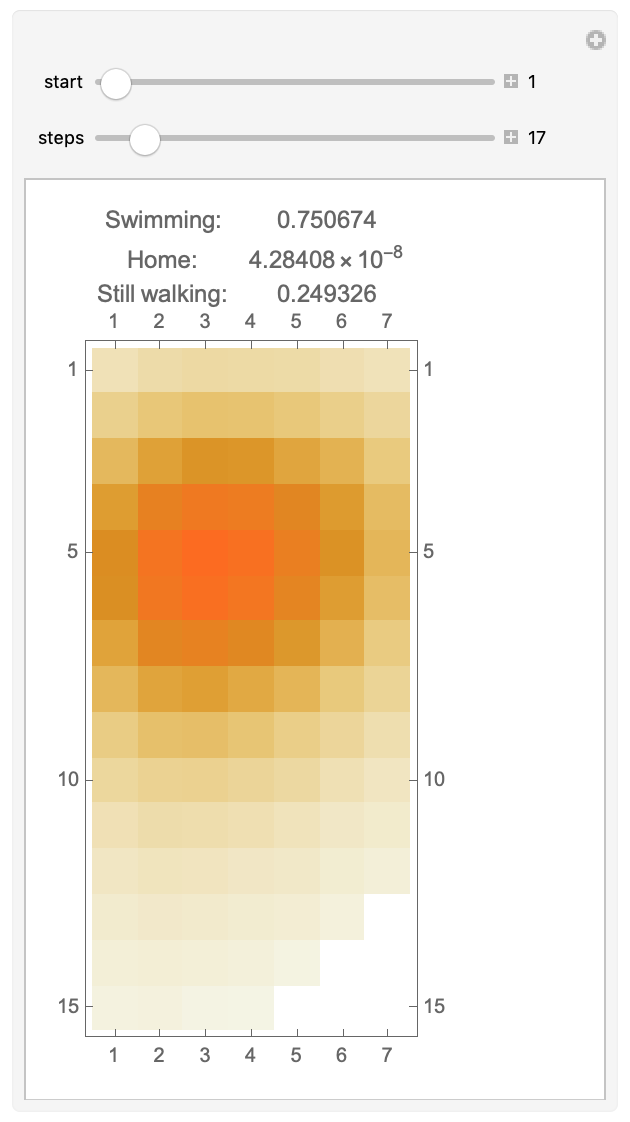So now if we find lim x→∞ Mx then we have the probability that he will make it at some point. I am just going to assume that if he hasn’t made it in 200 steps, then he will probably fall asleep on the plank anyway! So here are the probabilities that he is swimming or home after 200 steps: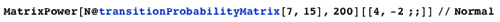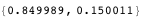In fact, 200 steps is enough to converge to 16 decimal places with this method, making it meaningless to go further in machine arithmetic. Of course in Mathematica, unlike my old Fortran 77 code, we are not limited to machine arithmetic, so here is the exact result for 500 steps: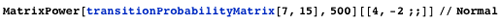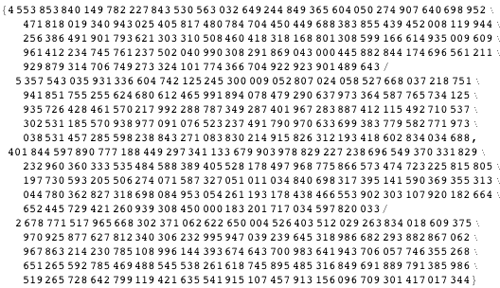This has converged to around 55 decimal places; here are the first 50: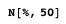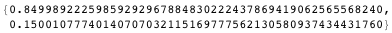Modeling drunken swimming is a harder problem, which I may revisit when Mathematica can do computational fluid dynamics (CFD).